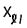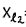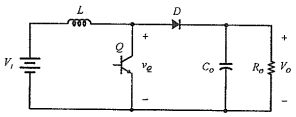milk26364325>試卷(2021/09/15)

# 110 年 - 110 國立臺灣大學_碩士班招生考試_電機工程研究所乙組:電力工程(含電力系統、電機機械、電力電子)#101255

【非選題】
1.

1. A 380 V, 20HP, 60 Hz four-pole Y-connected three phase induction motor has the following impedances in ohms per phase referred to the stator circuit: R1 =0.6 ohm, Xl = 1 ohm, R2=0.3 ohm, X2 =0.45 ohm,(magnetizing reactance) =25 ohm.
The total rotational losses are 1000W and are assumed to be constant. The core loss is neglected. For a rotor slip of 2 percent at the rated voltage and rated frequency, find the motor's stator current. (10%)

【非選題】
2.

2. The synchronous reactance for a round rotor synchronous generator is 1.0 and the armature resistance is negligible. Find the real power delivered by the generator when the terminal voltage isand the open-circuit voltage is(10%)

【非選題】
3.

3. The resistance and leakage reactance for the high voltage winding of a 25 KVA, 2000: 200V, 60 Hz, single phase transformer are R1 = 0.6 ohm and= 1.8 ohm, respectively . On the other hand, the resistance and leakage reactance for the low voltage winding are R2 = 0.006 ohm and= 0.018 ohm, respectively. Neglect the exciting current of the transformer. Select a base of 25 KVA and 2000 V for the high voltage winding.

【題組】(a) Compute the per unit values for the resistances and leakage reactance for the two windings. (10%)

【非選題】
4.【題組】(b) When the low voltage winding delivers power to a load of 20KW, 0.9 power factor lagging at 200V, find the per unit voltage at the high voltage side. (6%)

【非選題】
5.

4. Given the 60 Hz, balanced three-phase system shown below, findwhen(10%)【非選題】
6.

5. Assume tha. Find the maximum value for(10%)【非選題】
7.

6. The radius of each sub-conductor is 1 cm for the following 345 KV, 60 Hz, completely transposed balanced three phase lines. Find the per phase inductance In H/m and per phase capacitance to neutral in F/m. (10%)【非選題】
8.

7.An ideal boost converter operates at steady-state has the following specifications: VI=12 V, output voltage Vo =24 V, Cis very large, Switching frequency fs =1 00 kHz, Output power Po = 120 W.【題組】(a) How many modes if the converter operates in continuous conduction mode (CCM)? Draw the equivalent cireuit of each mode. (4%)

【非選題】
9.【題組】(b) Determine the inductance of L if the co nverter is operating at the boundary conduction mode (BCM), where inductor current touches zero right before switch turn-on. (4%)

【非選題】
10.【題組】(c) Follow qucestion (b). Draw the time waveforms of below variables for two switching periods. Mark the peak and valley values. Define the variable polarity in the circuit. Calculation procedure is required. Duty cycle, current through L, current through Co, voltage across L. (8%)

【非選題】
11.
8.Answer below questions. Explanation or mathematic derivations are required.

【題組】

(a) What is the de voltage across Clwhen the converter below is in steady-state operation? (5%)【非選題】
12.【題組】(b) Find the RMS value of a sine wave signal with peak value of 10 V. (3%)

【非選題】
13.【題組】

(c) Why the diode Dlin the buck converter below will turn-on after Ql tumn-of!? (3%)【非選題】
14.【題組】(d) Why the integration of inductor r voltage across a switching period is zero for a buck converter at steady-state? (3%)

【非選題】
15.【題組】(e) Follow (c). Assume there is a small inductor in series with RL. Draw the voltage waveform of this inductor voltage for two switching periods. (4%)

### 懸賞詳解

#### 國一地理上第三次

28.( ) 下列哪些是造成臺灣近年水資源日趨不足的因素？ (甲)人口密度高(乙)植被種類多樣(丙)水利設施不足(丁)坡 陡、流急(戊)降雨時間分布不均 (A)甲�...